×#### Thank you for registering.

One of our academic counsellors will contact you within 1 working day.

Click to Chat

1800-1023-196

+91-120-4616500

CART 0

• 0

MY CART (5)

Use Coupon: CART20 and get 20% off on all online Study Material

ITEM
DETAILS
MRP
DISCOUNT
FINAL PRICE
Total Price: Rs.

There are no items in this cart.
Continue Shopping• Complete JEE Main/Advanced Course and Test Series
• OFFERED PRICE: Rs. 15,900
• View Details

```Mensuration III (Surface Area and Volume of a Right Circular Cylinder) Exercise 22.1

Question: 1

Find the curved surface area and total surface area of a cylinder, the diameter of whose base is 7 cm and height is 60 cm.

Solution: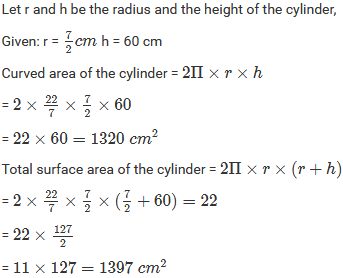Question: 2

The curved surface area of a cylindrical rod is 132 cm2. Find its length if the radius is 0.35 cm.

Solution:Question: 3

The area of the base of a right circular cylinder is 616 cm2 and its height is 2.5 cm. Find the curved surface area of the cylinder.

Solution: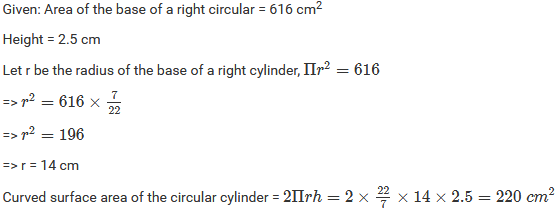Question: 4

The circumference of the base of a cylinder is 88 cm and its height is 15 cm. Find its curved surface area and total surface area.

Solution:

Given: Height, h = 15 cm

Circumference of the base of the cylinder = 88 cm2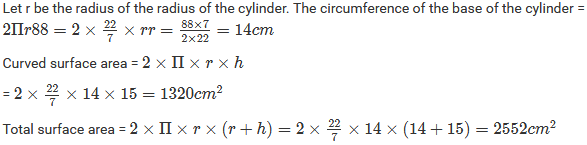Question: 5

A rectangular strip 25 cm x 7 cm is rotated on the longer side. Find the total surface area of the solid thus generated.

Solution:

Since the rectangle strip of 25 cm x 7 cm is rotated about the longer side, we have:

Height, h = 25 cm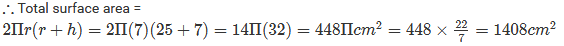Question: 6

A rectangular sheet of paper, 44 cm x 20 cm, is rolled along its length to form a cylinder. Find the total surface area of the cylinder thus generated.

Solution:

The rectangular sheet of paper 44 cm x 20 cm is rolled along its length to from a cylinder. The height of the cylinder is 20 cm and circumference is 44 cm.Question: 7

The radii of two cylinders are in the ratio 2 : 3 and their heights are in the ratio 5 : 3. Calculate the ratio of their curved surface areas.

Solution: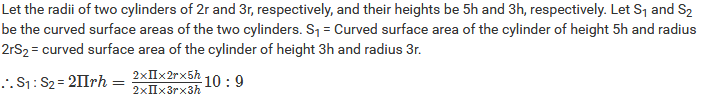Question: 8

The ratio between the curved surface area and the total surface area of a right circular cylinder is 1:2. Prove that its height and radius are equal.

Solution: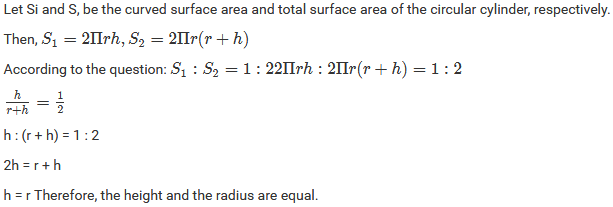Question: 9

The curved surface area of a cylinder is 1320 cm2 and its base has diameter 21 cm. Find the height of the cylinder.

Solution: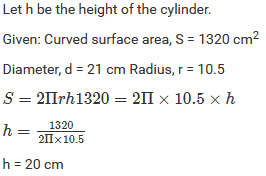Question: 10

The height of a right circular cylinder is 10.5 cm. If three times the sum of the areas of its two circular faces is twice the area of the curved surface area. Find the radius of its base

Solution: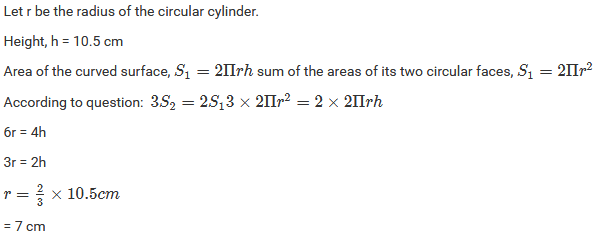Question: 11

Find the cost of plastering the inner surface of a well at Its 9.50 per m2, if it is 21 m deep and diameter of its top is 6 m.

Solution: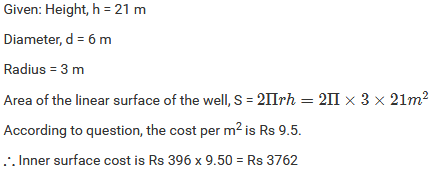Question: 12

A cylindrical vessel open at the top has diameter 20 cm and height 14 cm. Find the cost of tin-plating it on the inside at the rate of 50 paise per hundred square centimetres.

Solution: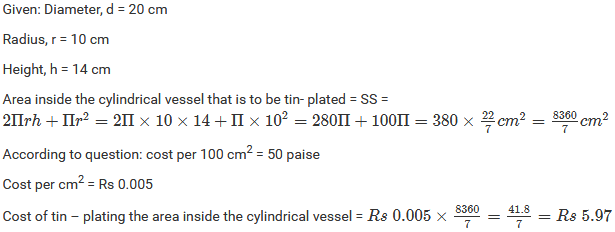Question: 13

The inner diameter of a circular well is 3.5 m. It is 10 m deep. Find the cost of plastering its inner curved surface at Rs 4 per square meter.

Solution: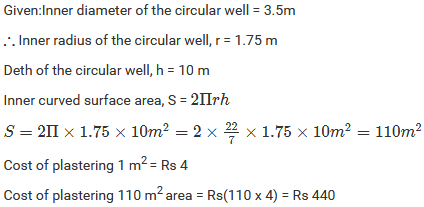Question: 14

The diameter of a roller is 84 cm and its length is 120 cm. It takes 500 complete revolutions moving once over to level a playground. What is the area of the playground?

Solution: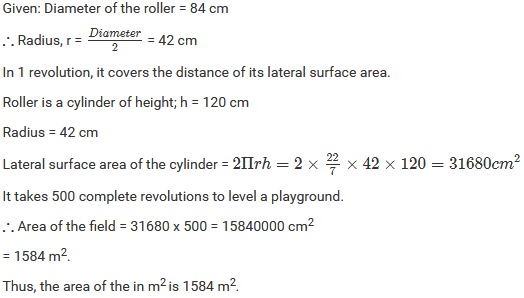Question: 15

Twenty-one cylindrical pillars of the Parliament House are to be cleaned. If the diameter of each pillar is 0.50 m and height is 4 m, what will be the cost of cleaning them at the rate of Rs 2.50 per square meter?

Solution:Question: 16

The total surface area of a hollow cylinder which is open from both sides is 4620 sq. cm, the area of the base ring is 115.5 sq. cm and height 7 cm. Find the thickness of the cylinder.

Solution: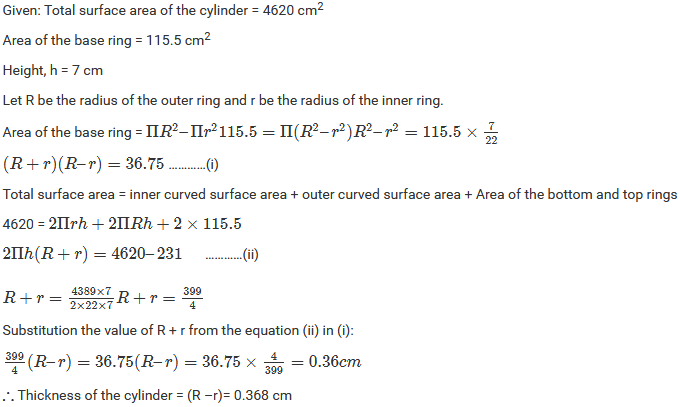Question: 17

The sum of the radius of the base and height of a solid cylinder is 37 m. If the total surface area of the solid cylinder is 1628 m2, find the circumference of its base.

Solution:

Let r and h be the radius and height of the solid cylinder.

Given: r + h = 37 m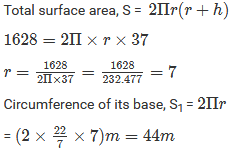Question: 18

Find the ratio between the total surface area of a cylinder to its curved surface area, given that its height and radius are 7.5 cm and 3.5 cm.

Solution: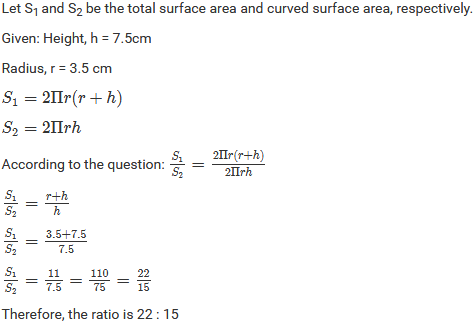Question: 19

A cylindrical vessel, without lid, has to be tin-coated on its both sides. If the radius of the base is 70 cm and its height is 1.4 m, calculate the cost of tin-coating at the rate of Rs 3.50 per 1000 cm2.

Solution:```### Course Features

• 728 Video Lectures
• Revision Notes
• Previous Year Papers
• Mind Map
• Study Planner
• NCERT Solutions
• Discussion Forum
• Test paper with Video Solution